2022: SklogWiki celebrates 15 years on-line

# Difference between revisions of "E3B water model"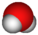This article is a 'stub' about water and/or ice. It has no, or next to no, content. It is here at the moment to help form part of the structure of SklogWiki. If you add material to this article, remove the {{Stub-water}} template from this page.

The E3B (effective three-body) model of water.

## Parameters

The E3B potential is given by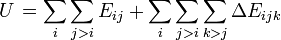$\left. U \right.= \sum_i \sum_{j>i} E_{ij} + \sum_i \sum_{j>i}\sum_{k>j} \Delta E_{ijk}$

where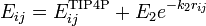$E_{ij} = E_{ij}^{\mathrm{TIP4P}} + E_2 e^{-k_2r_{ij}}$

where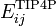$E_{ij}^{\mathrm{TIP4P}}$ represents the TIP4P model of water

and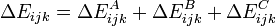$\Delta E_{ijk} =\Delta E_{ijk}^A + \Delta E_{ijk}^B + \Delta E_{ijk}^C$

where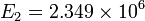$E_2 = 2.349\times10^6$ kJ/mol,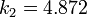$k_2 = 4.872$ Å-1,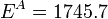$E^A = 1745.7$ kJ/mol,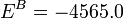$E^B = -4565.0$ kJ/mol, and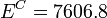$E^C = 7606.8$ kJ/mol.